### Home > CALC > Chapter Ch3 > Lesson 3.2.3 > Problem3-80

3-80.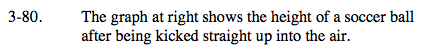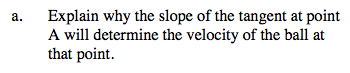Since the slope of a secant determines the Average Velocity (between two points),the slope of a tangent will determine the Instantaneous Velocity (at one point).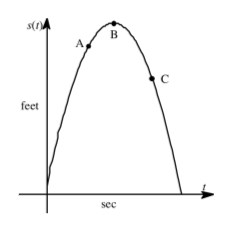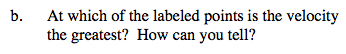Be sure to consider the difference between velocity and speed.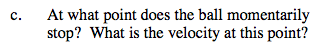Since velocity changes from positive to negative continuously, and since 0 is in between positive and negative values, the Intermediate Value Theorem guarantees that velocity will (at one moment) be 0; therefore, the ball will momentarily stop.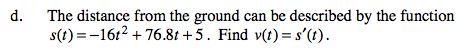Use the Power rule.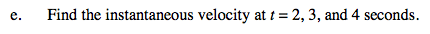Use your derivative function in part (d) to find v(2), v(3) and v(4).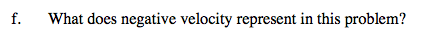What is happening to the soccer ball when the velocity is negative?